Mobile QR Code1. (Department of the Electronics and Robotics Engineering, Busan University of Foreign Studies, Busan, Korea kyoojae@bufs.ac.kr )

Thermal characteristics, Phase change materials, Electric vehicle, Battery pack and thermal control

1. Introduction

Electric vehicles are actively being researched and commercialized in each country due to global warming caused by fossil fuels . The battery pack is an important energy source that determines the mileage of an electric vehicle and is sensitively affected by the external air temperature . In other words, the mileage decreases under sub-zero temperature conditions in winter, and thermal runaway has a risk of fire or explosion at high temperature conditions in summer . Therefore, the optimal temperature control of the battery pack is a very important factor, and the temperature control of the battery pack using a PCM has been promoted by many researchers.

Research on a battery pack under the conditions of sub-zero air temperature was conducted using the air-cooling method by Peng et al. . Research on a battery pack under high temperature conditions was conducted by using water cooling and air cooling by Al-Zareer . These systems have disadvantages such as increased vehicle weight, installation complexity, and maintenance issues .

In general, the thermal energy of an electric vehicle discharges high-temperature heat energy from the driving motor and battery pack under charging and discharging conditions. Cooling heat is produced for the driving motor and battery pack by using a chiller in summer.

Shin et al. did research and experimental studies and reported that PCM with high thermal conductivity is the most suitable material for maintaining the temperature of a battery pack. The material can be used by storing and optimally distributing heat generated from the battery pack . Jiang et al. demonstrated that the heat transfer characteristic of PCM increases as the operating time of the battery increases and that PCM cooling transfers heat more effectively compared to other cooling methods . PCM is a material that guarantees the thermal stability of the battery pack. It is flexible, permanent, easy to install, and has the advantage of minimizing the weight added to the vehicle without an external power supply and maintenance .

Zaho et al.  concluded that after conducting experiments on traditional methods and PCM, PCMs more effectively reduce battery temperature than other methods. Karimi et al.  conducted experiments on a li-ion battery with PCMs and observed that battery temperature was reduced by about 70% when compared to one without PCMs. From the above literature, the motivations for this study are described below:

$\color{color-4}{·}$ Due to more advantages with PCM, it is clear that PCM is the best substitute when compared with other traditional methods.

$\color{color-4}{·}$ Much research was carried out on a li-ion battery, but there is scarce research on temperature changes in a lithium polymer battery. Hence, in this research, we selected a lithium polymer battery as a research subject.

$\color{color-4}{·}$ Many researchers have focused their research on cooling of the battery, but very little research is available on warming of the battery during winter season in cold climatic conditions. In this study, we focused on warming of the battery at low temperatures.

$\color{color-4}{·}$ No research work has been carried out on RT15, RT31, EG26, and EG28 PCMs. In this study, we used these PCMs.

The novelties in this paper are:

$\color{color-4}{·}$ Heat transfer and thermal energy storage characteri-stics of four PCMs such as RT15, RT31, EG26, and EG28 are analyzed from solid to liquid phase when heated and from liquid to solid phase when cooled.

$\color{color-4}{·}$ The thermal properties of the one-cycle phase trans-formation from liquid to solid were analyzed.

$\color{color-4}{·}$ Thermal conduction and thermal storage tests were performed on four PCMs in the environment of -20 to 60$^{\circ}$C.

$\color{color-4}{·}$ In order to confirm the hysteresis of thermal pro-perties, expanded graphite PCM was analyzed and applied in order to perform optimal temperature control of the battery pack.

$\color{color-4}{·}$ A BTMS was designed and manufactured using the thermal characteristics of PCM and an optimized hysteresis PI control method, and the performance of the proposed temperature control system was verified through experiments.

2. Modeling of Battery Pack with PCM Thermal Characteristics

In a battery pack, as electrons move during the charging and discharging process, thermal energy is generated in the battery pack. In addition, this heat is in thermal equilibrium in the process of transferring heat to the atmosphere together with the PCM of the battery. The battery pack utilizes this thermal equilibrium principle to control heat by optimizing the operating temperature range of the battery pack by utilizing the cold and hot latent heat energy stored in the PCM. In this state, the equation for the amount of heat generated from the battery pack when the electric vehicle is charging or discharging is Eq. (1) .

(1)
$q_{\textit{battery}}\left(t\right)=I\left(U-V-T\frac{du}{dt}\right)$

where

$~ q_{\textit{battery}}\left(t\right)$ : Heat generated by battery [J]

$I\left(U\hbox{--}V\right)$ : Heating potential

$IT$ : Entropy coefficient

This thermal energy is absorbed by the PCM combined with the battery pack, and the equation for thermal energy transferred from the battery pack to the PCM at this time is Eq. (2).

(2)
$q_{pcm}\left(t\right)=hA\left(T-T_{a}\right)+mc_{p}\frac{du}{dT}$

where

[$h$]: Heat transfer coefficient per battery [Kwm/k]

[$A$]: Cross-sectional area of battery [mm]

$\textit{T}$ : Initial Battery Temperature [K]

$T_{a}$ : Ambient temperature [K]

[$c_{p}$]: Specific heat [J/kg K]

[$\textit{m}$]: Battery mass [Kg]

The battery pack generates heat through electron transfer during charging and discharging, and this heat will be balanced by the amount of PCM and convective heat transfer. The PCM is additionally placed around the battery pack to safely maintain the thermal state of the battery pack and to manage thermal control.

When the vehicle is stopped or parked for a long time, the amount of thermal energy transferred from the PCM to the battery pack is given in Eq. (3).

(3)
$hA\left(T-T_{a}\right)+m_{p}c_{p}\left(\frac{du}{dT}\right)_{p}+m_{b}c_{b}\left(\frac{du}{dT}\right)_{b}=0$

Thermal energy may be transferred or supplied from the PCM while the battery is in operation or inactive. The safe operating temperature range of the battery pack is 25 to 40$^{\circ}$C.

PCM must have excellent thermal conductivity and thermal storage properties in order to achieve thermal equilibrium for the battery pack under optimal temperature conditions [12,13]. For example, in extreme conditions below zero, an electric vehicle must store thermal energy of a battery pack generated during driving in a PCM and then supply high-temperature thermal energy to the battery pack when parking and driving. Additionally, in high temperature conditions above 40$^{\circ}$C, the vehicle's cooler operates to cool the battery pack and stores the cooling heat energy in a PCM to provide cooling heat to the battery pack while the vehicle is parked and driven in high temperature conditions.

PCM can be installed very quickly and easily in an external form without using a power source, and the material guarantees the thermal stability of the battery pack. In this study, heat conduction and thermal storage characteristic tests were performed on four PCMs in an environment of -20 to 60$^{\circ}$C. Hysteresis of thermal characteristics was analyzed for expanded graphite PCMs EG26 and EG28, which have the best thermal characteristics. The hysteresis measured through the experiment can predict the latent heat or heat storage amount of the PCM, and the temperature threshold of the PCM for heat energy transfer of the battery pack can be known.

PCM is in a solid state at low temperatures and begins to melt when heated, and when the temperature is continuously increased, PCM is completely transformed into a liquid state. The PCM performance curve is shown in the graph below in Fig. 1. Conversely, if the temperature is low, the PCM is solidified in a liquid state, and if the temperature is continuously low, the PCM becomes a completely solid state. The temperature characteristic curve of PCM shows different inclined curves when the temperature rises and falls. The temperature deviation value of this curve is called latent heat, and this latent heat absorbs or releases high temperature and cooling energy in the process of repeated melting and solidification.

(4)
$q_{\textit{Heating}}=~ \pm \int mc_{p}\Delta T_{\textit{heating}}dt$

where

$q_{\textit{Heating}}$ : heat generated in battery operation

[$\textit{m}$]: Mass of battery [kg]

[$c_{p}$]: Specific heat in [J/kg K]

$\Delta T_{\textit{heating}}$ : Temperature difference [K]

The hysteresis curve of PCM in Fig. 1 is used to control the on/off switch based on a PID controller. In this paper, the hysteresis curve of thermal energy was derived as shown in Fig. 6 through experiments on the thermal characteristics of the solid-to-liquid and solid-to-liquid phase transformation cycles of PCM EG26 and EG28. The amount of latent heat storage capacity with this hysteresis is given by Eq. (4) for heating and cooling conditions of PCM.

Fig. 1. Thermal Hysteresis Evaluation Model.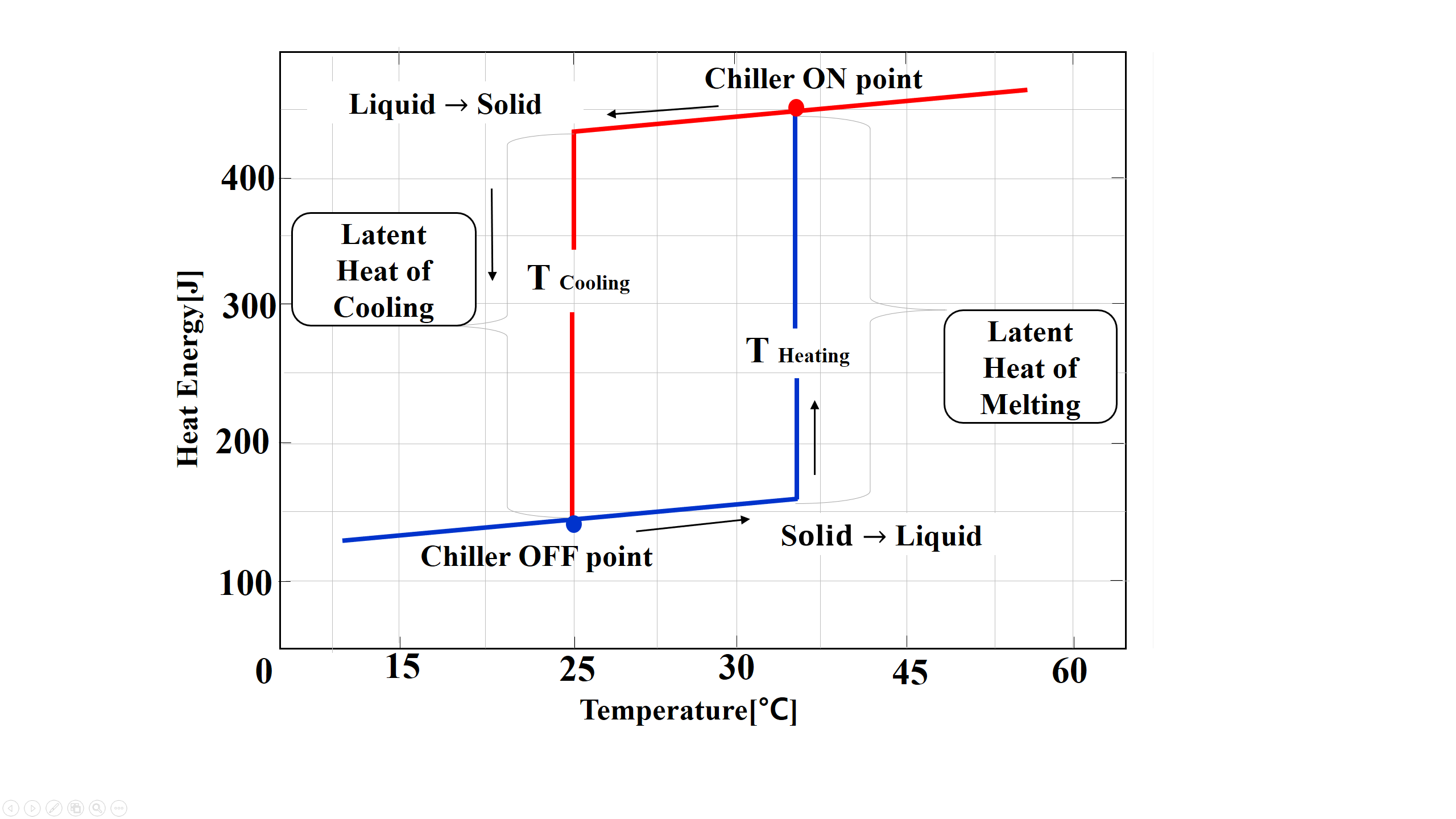3. Design of Feedback Controller with PCM Hysteresis Thermal Characteristics

The configuration of the thermal management control system of the battery pack with PCM proposed in this paper is shown in Fig. 2. The optimal temperature condition is set as a command value, and the measured temperature value of the battery pack is fed back to calculate an error value. This error value is compensated by the PI controller, and the on/off state is determined by the PCM hysteresis thermal characteristic to determine the operating state of the chiller to control the high temperature and cooling heat of the PCM. It controls the optimum temperature of the battery pack.

The reduced transfer functions in Fig. 2 are obtained by each component in the controller. The outlet PCM temperature is given as feedback controller temperature input. After error correction, this input temperature is given as input for the PI controller. The controller equation for PI is represented as $g_{P}\left(t\right)$ in Eq. (5).

(5)
$g_{P}\left(t\right)=k_{c}e\left(t\right)+\frac{1}{T_{i}}~ \int e\left(t\right)dt~$

The proposed model in Fig. 2 implements a temperature controller with a very slow response of the thermodynamic system. The PI controller compensates for the temperature deviation value and controls the chiller operation (on/off) based on the state curve of the hysteresis controller. At this time, the dynamic control of the chiller uses Eq. (6).

(6)
$g_{H}\left(t\right)=\sum _{T_{min}}^{T_{max}}B\left(t\right)dt~$

where $B\left(t\right)$ is the temperature recorded by the PCMs during operating condition of the battery.

The battery electric potential state of heat generated due to charging and discharging condition is given as $g_{Bat}\left(t\right)$ and is shown in Eq. (7),

(7)
$$g_{\text {Bat }}(t)=\frac{d u}{d t} I U-\frac{d u}{d t} I V-\frac{d u}{d t}\left(I T \frac{d u}{d t}\right)$$

Considering the load condition, the battery’s dynamic equation can be obtained as an approximate model as in Eq. (8). Also, the dynamic equation in which the generated thermal energy is transferred to the PCM is Eq. (9). The reduced form of the transfer function of the battery and PCM is given as $G_{B}\left(s\right),$ as shown in Eq. (10).

(8)
$G_{B}\left(s\right)=\frac{aeS^{3}+\left(bf+cg+dh\right)S^{2}+\left(i+j+k\right)S+1}{eS^{3}}$

where

$a=R_{1}R_{2}R_{3}$, $b=R_{1}R_{2}$, $c=R_{1}R_{3}$

$d=R_{2}R_{3}$, $\mathrm{e}=C_{1}C_{2}C_{3}$, $f=C_{1}C_{2}$

$g=C_{1}C_{3}$, $h=C_{2}C_{3}$, $i=R_{1}C_{1}$

$j=R_{2}C_{2}$, $k=R_{3}C_{3}$

(9)
$g_{pcm}\left(t\right)=hA\left(T-T_{a}\right)+mC_{p}\frac{du}{dt}~$
(10)
$G_{B}\left(s\right)=\frac{G_{Bat}\left(s\right)}{G_{pcm}\left(s\right)}$

The reduced transfer function $G_{O}\left(s\right)$ of the entire system can be expressed as the temperature output value of the battery pack with respect to the temperature control command value as in Eq. (10), and the transfer function at this time is as Eq. (11).

(11)
$G_{O}\left(s\right)=\frac{Y\left(s\right)}{X\left(s\right)}=\frac{G_{P}\left(s\right)G_{H}\left(s\right)G_{Bat}\left(s\right)G_{B}\left(s\right)}{1+G_{P}\left(s\right)G_{H}\left(s\right)G_{Bat}\left(s\right)G_{B}\left(s\right)}$

where

$G_{P}\left(s\right)$ : PI controller equation

$G_{H}\left(s\right)$ : Hysteresis equation to controller

$G_{Bat}\left(s\right)$ : Battery equation

$G_{B}\left(s\right)$ : Reduce battery and PCM equation

$G_{O}\left(s\right)$ : Controller output

$T_{o} {\quad}$: Final PCM temperature$~ \left[K\right]$

$T_{i} {\quad}$: Initial PCM temperature $\left[K\right]$

By using the temperature control in Fig. 3 and Eq. (10) with PI control, as suggested in this paper, the expanded graphite PCM can stably under-control the battery temperature within the range of 28 to 34$^{\circ}$C. The cooler can control the heat of the PCM by using an air-cooled cooling method. The proposed control method in Fig. 3 was implemented using a PIC 18 series microcomputer.

Fig. 3. Battery Pack: (a) Design, (b) H/W Realization.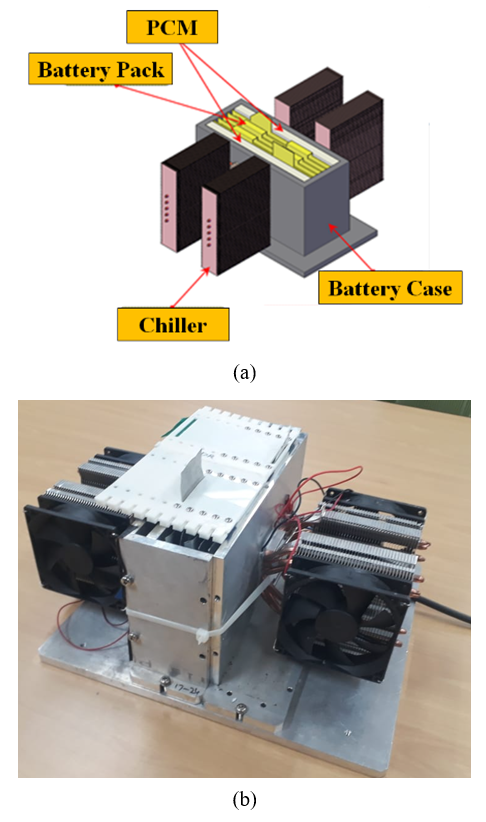Fig. 4. Thermal Controller using PIC18F8720 MICOM.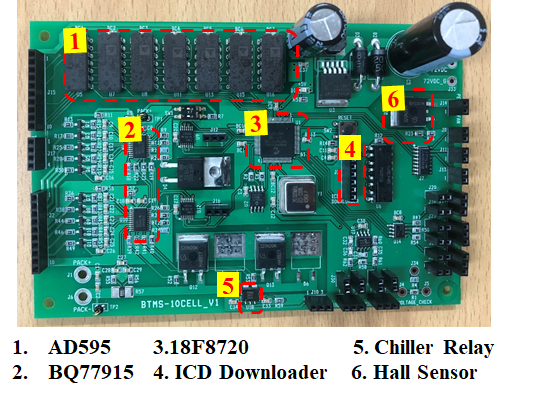4. Experimental Results

4.1 Experiment Setup

To verify the proposed control method in Fig. 3, a battery pack system was designed as shown in Fig. 3(a). Five lithium polymer pouch batteries were connected in series, and a PCM was installed outside the battery pack. A chiller was installed outside the battery pack for thermal control and measurement, and a thermocouple sensor was installed in each cell to measure the temperature change during charging and discharging of the lithium polymer battery. as shown in Fig. 3(b).

Also, to perform the proposed thermal feedback control in Fig. 3 and Eq. (10), a circuit was designed using a PIC MICOM, as shown in Fig. 4. The designed battery thermal management controller can control the PCM temperature for melting and solidification as the battery temperature rises and falls. The designed thermal controller consists of a temperature sensor (AD595), a BQ77915 for battery cell charging and discharging control, a current and voltage sensor, and a switching circuit to control the chiller. This controller board controls the voltage of the battery pack during charging and discharging of the electric vehicle battery pack and also performs temperature control using PCM.

Table 1. Physical parameters of RT15, RT31, EG26, and EG28.
 Parameters RT15 RT31 EG26 EG28 Density [kg/m3] 750 880 850 500 Heat storage capacity [kJ/kg] 155 165 140 160 Thermal conductivity [w/mK] 2.0 2.0 2.2 2.5 Melting point [°C] 15 31 26 28
Table 2. Temperature comparison of battery at 25$^{\circ}$C, 60$^{\circ}$C, and 0$^{\circ}$C.
 PCM Battery Temp with no PCM[°C] Battery Temp with PCM[°C] 25 to 25 25 to 60 -10 to 0 25 to 25 25 to 60 -10 to 0 RT15 37 49 -4 31 45 -6 RT31 37 49 -4 30 40 -3.5 EG28 37 49 -4 27 36 -1.8 EG26 37 49 -4 31 37.5 -2.0
Fig. 5. PCM Characteristic Evaluation Setup.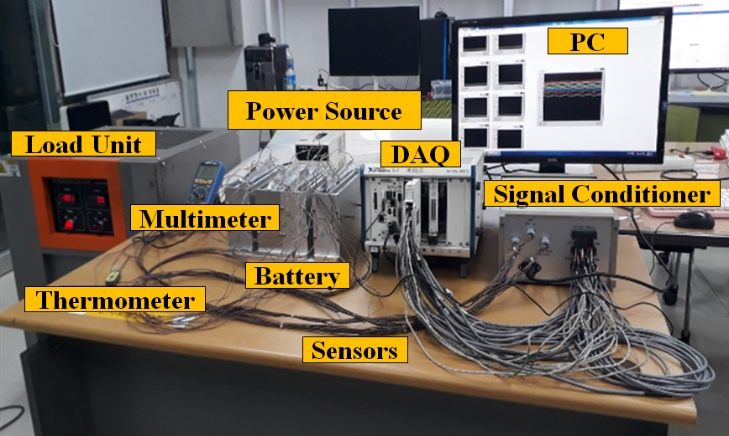Fig. 6. Hysteresis Evaluation from PCM Properties.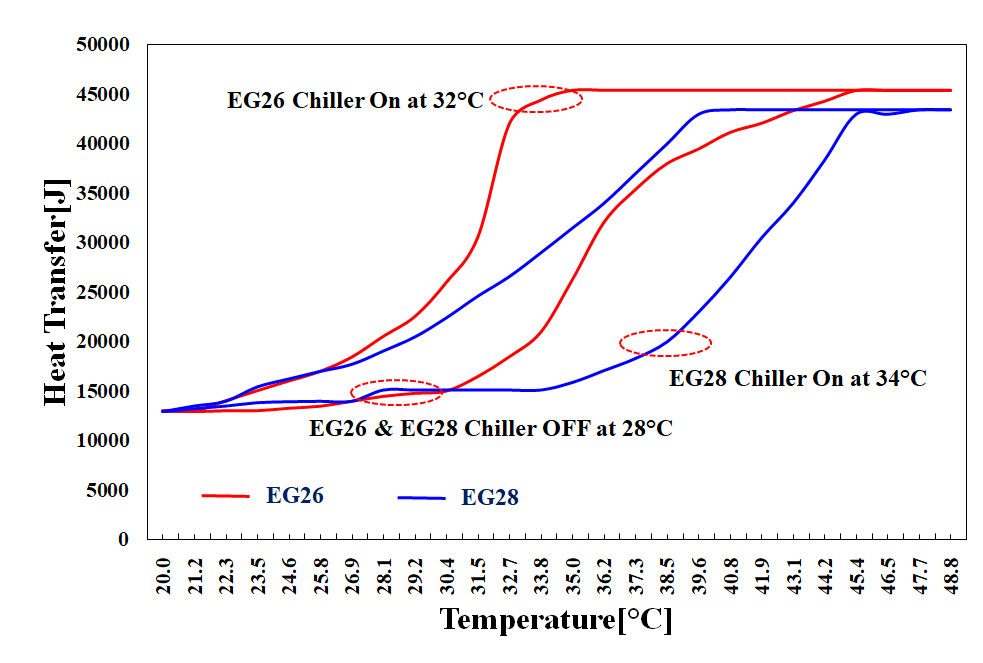4.2 PCM Thermal Performance Test

In order to evaluate the heat storage, melting, and solidification capacity of the PCM under extreme temperature conditions, it was constructed as shown in Fig. 5. Thermal conductivity test subjects were installed in the battery module with 2 types of paraffin-based PCM, RT15 and RT31, and expanded graphite-based EG26 and EG28. These heat conduction and heat control experiments consist of a thermal measuring device (DAQ), a signal conditioner, a computer, and a resistive load device.

In this experimental method, the experiment is performed under three conditions. The battery is first heated to room temperature (25$^{\circ}$C) and then heated from 25 to 60$^{\circ}$C. Third, we heat the temperature environment to 0$^{\circ}$C with the battery pack exposed to -10$^{\circ}$C. We performed the experiment in a controlled environment for 6 hours. All batteries were initially charged to 4.0V, paused for 30 minutes, and then periodically discharged to 2.6V to measure heat transfer to the PCM. For these experimental conditions, repeated experiments were initially performed at room temperature (25$^{\circ}$C), high temperature (60$^{\circ}$C), and extreme conditions (-20$^{\circ}$C).

As PCMs applied in this experiment, paraffin-based RT15 and RT31 and graphite diffusion series EG26 and EG28 were used, and the physical parameters of these PCMs are shown in Table 1. Table 2 shows the results of measuring the change in temperature characteristics of a battery module to which PCM is applied and a battery module to which PCM is not applied in the experimental apparatus of Fig. 6. The thermal properties of the selected PCM can be easily confirmed from the experimental results.

For the battery module without PCM, the battery temperatures of RT15, RT31, EG26, and EG28 were 37$^{\circ}$C, 49$^{\circ}$C, and -4$^{\circ}$C at 25$^{\circ}$C, 60$^{\circ}$C, and 0$^{\circ}$C, respectively. For RT15, RT31, EG26, and EG28, each battery temperature was controlled differently, as shown in Table 2 according to the latent heat temperature characteristics of PCM. In particular, it was confirmed that EG28 satisfies the optimum temperature conditions for battery cells at 27$^{\circ}$C, 36$^{\circ}$C, and -1.8$^{\circ}$C under the experimental conditions. At the hot temperature condition of 60$^{\circ}$C, the battery with no PCMs was 49$^{\circ}$C, but with PCMs, the battery temperature was recorded as 45$^{\circ}$C, 40$^{\circ}$C, 36$^{\circ}$C, and 37.5$^{\circ}$C, respectively.

In the extreme cold condition, the battery temperature with no PCMs was -4$^{\circ}$C, and with PCMs, temperatures were -6$^{\circ}$C, -3.5$^{\circ}$C, -1.8$^{\circ}$C, and -2.0$^{\circ}$C, respectively. Also, high thermal gradients were observed in the battery with the PCMs and battery with no PCMs. At room temperatures, ${\Delta}$T was 10$^{\circ}$C. At 60$^{\circ}$C, ${\Delta}$T was 7$^{\circ}$C, and at extreme cold climatic conditions, the thermal gradient ${\Delta}$T was 2$^{\circ}$C.

In order to perform optimal temperature control of the battery pack, a battery pack temperature control system (BTMS) was designed using a hysteresis PID control method that considers the thermal characteristics of the PCM shown in Fig. 2. PCM stores high heat when it changes from solid state to liquid state based on the phase change point, and PCM stores cooling heat when the phase changes from liquid state to solid state. In this study, the optimum melting point and optimum solidification temperature were observed for the selected PCM by obtaining the low melting point of each PCM, and a hysteresis curve was derived based on the characteristics. As a result of evaluating the optimum temperature for the Rubitherm PCM of EG26 and EG28, it was observed that the battery temperature could be controlled to the optimum temperature condition due to the thermal latent heat characteristics of the PCMs.

Therefore, in order to properly control the heat energy absorption and emission of the PCM for optimal temperature control of the battery, the microcontroller uses the chiller of the cooling device to properly control the temperature of the battery pack with the PCM. Expanded graphite PCMs EG26 and EG28 have different thermal properties when heated from 20$^{\circ}$C to 50$^{\circ}$C, as shown in Fig. 6.

The physical parameters of extended graphite PCM EG26 and EG28 are shown in Table 1. As shown in Fig. 6, it can be seen that the phases of EG26 and EG28 are transformed by different phase shift curves. The experimental results of Fig. 6 are summarized as follows. The phase of EG26 changes to liquid at 32.7$^{\circ}$C, and EG26 changes at 40.0$^{\circ}$C by raising the temperature from 20$^{\circ}$C to 50$^{\circ}$C and then lowering it to 50$^{\circ}$C to 20$^{\circ}$C. PCM also stores potential thermal energy during the solid-to-liquid and liquid-to-solid phase transitions. The latent heat section is 30$^{\circ}$C to 35$^{\circ}$C for EG26 and 30$^{\circ}$C to 40$^{\circ}$C for EG28. The operating range of the battery pack is optimized from 25$^{\circ}$C to 40$^{\circ}$C, making the EG28 with a wide latent heat section more suitable for optimum temperature control.

The temperature control of the battery pack uses these physical temperature characteristics to control the operation of the chiller. In the thermal management control, the chiller is turned off at 32$^{\circ}$C for EG26 and 34$^{\circ}$C for EG28 in the rising curve, where the temperature gradually increases when heat is transferred from the battery pack to the PCM. Also, when the battery pack is cooled from 50$^{\circ}$C to 20$^{\circ}$C, the EG26 and EG28 have a descending curve in which the temperature decreases, and the chiller turns off at 20$^{\circ}$C. In this way, the chiller of the battery pack with PCM can be controlled.

Fig. 7. Experimental Result of Battery Pack Temperature: (a) Initial battery temp=25$^{\circ}$C, Chamber temp=25$^{\circ}$C, (b) Initial battery temp=25$^{\circ}$C, Chamber temp=45$^{\circ}$C, (c) Initial battery temp=-10$^{\circ}$C, Chamber temp=0$^{\circ}$C.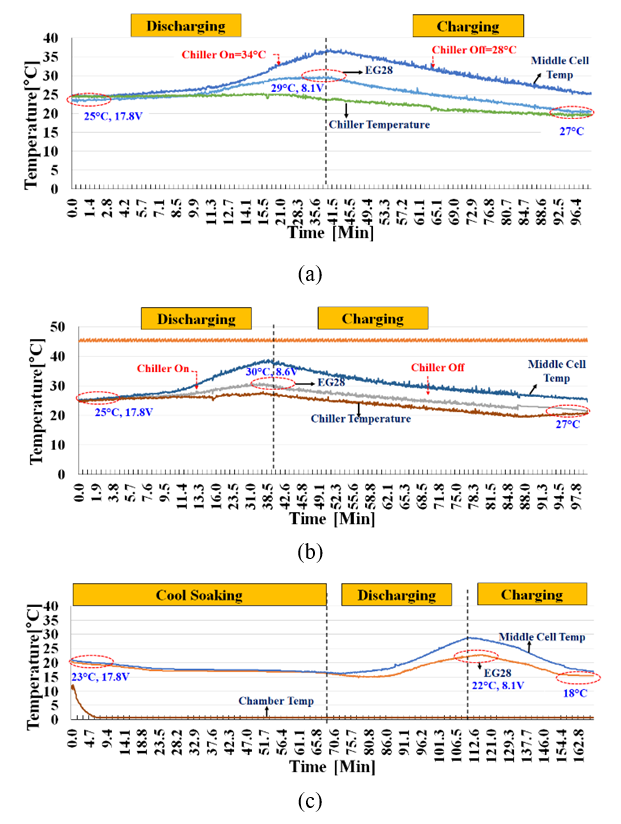4.3 Experiment Results and Discussion

To verify the performance of the proposed experimental model, the experimental device was configured as shown in Fig. 5, and the temperature control of the battery pack was implemented using the algorithm shown in Fig. 2. The control condition in Fig. 2 applied at this time is the same as that of EG 28, and the chiller configures the controller to be off at 28$^{\circ}$C and on at 34$^{\circ}$C. In addition, the charging/discharging of the batteries was performed by charging each battery voltage to 3.6V, resting for 30 minutes, and then discharging the battery pack up to 40% while recording the battery pack temperature change.

In this experiment, as shown in Fig. 7, the thermal control performance experiment was studied for EG28 through a thermal sensor at 5 points under 3 temperature conditions. The first experimental condition is a graph of battery temperature that occurs when the chamber is 25$^{\circ}$C, and the initial temperature of the battery pack is 25$^{\circ}$C, as shown in Fig. 7(a), when discharging and charging for 60 minutes and 100 minutes. The temperature of the battery pack increases during discharging, the chiller operates when the battery pack temperature is 34$^{\circ}$C, and the chiller turns off when the battery pack temperature is 28$^{\circ}$C during charging.

In Fig. 7(b), when the temperature of the battery pack rises in the chamber at 45$^{\circ}$C, the chiller operates when the initial temperature of the battery pack is 34$^{\circ}$C, and the temperature of the battery pack decreases so that the battery pack temperature is 28$^{\circ}$C. The chiller is controlled off. At this time, the chiller was operated for about 70 minutes. In the third experiment, the battery temperature drops rapidly from the battery pack temperature of 25$^{\circ}$C to the chamber temperature of 0$^{\circ}$C, as shown in Fig. 7(c). At this time, the battery pack operates stably in the range of 15$^{\circ}$C to less than 30$^{\circ}$C by the latent heat energy of EG28. Through this experiment, the stable performance of the temperature control system of the battery pack to which the proposed PCM is applied was confirmed.

5. Conclusion

In this paper, we proposed a temperature control system for battery packs with EG28, an extended graphite PCM, as a method to control the optimal temperature of electric vehicle battery packs under extreme conditions. Even in extreme environments, the battery pack must be controlled to a safe temperature operating range of 25-40$^{\circ}$C. The battery pack proposed in this paper stores the thermal energy of the battery pack generated during driving in extreme conditions and PCM, and the battery pack will manage thermal energy during parking and driving.

In order to verify the temperature control performance, heat conduction and heat storage characteristic tests were performed on four PCMs in an environment of -20 to 60$^{\circ}$C. In order to perform optimal temperature control of the battery pack, a BTMS was designed and manufactured using the thermal characteristics of PCM and the optimized hysteresis PI control method shown in Figs. 3 and 4. The proposed temperature control system was tested as shown in Fig. 5.

From the results, it was observed that out of the four PCMs, expanded graphite PCMs performed better and controlled the battery temperature within safe operating conditions. After this, we analyzed EG28 and EG26 PCMs’ properties and applied them on the BTMS to control the chiller operating points. Through this, the proposed thermal control system yielded the best results.

ACKNOWLEDGMENTS

This work was supported by a research grant from the Busan University of Foreign Studies in 2021. It supported the funds to develop this work and also to attend a technical seminar. Finally, I would like to express my sincere gratitude to "Busan University of Foreign Studies."

REFERENCES

1
Angani Amarnathvarma, Kim Euisong, Shin Kyoojae, 2020, Improvement of Thermal Performance of Electric Vehicle Battery Pack with Phase-change Material., Sensors and Materials, Vol. 32, No. 5, pp. 1609-1622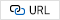2
Talluri T, Kim TH, Shin KJ., 2020 Jan., Analysis of a Battery Pack with a Phase Change Material for the Extreme Temperature Conditions of an Electrical Vehicle., Energies., Vol. 13, No. 3, pp. 5073
Peng Xiongbin, et al. , 2020, A Thermal Investigation and Optimization of an Air-Cooled Lithium-Ion Battery Pack., Energies, Vol. 13, No. 11, pp. 29564
Maan Al-Zareer , Ibrahim Dincer , Marc A.Rosen , 2019, A novel approach for performance improvement of liquid to vapor based battery cooling systems., Energy Conversion and Management, Vol. 187, pp. 191-2045
Rao Z., Wang S., 2011, A review of power battery thermal energy management., Renewable and Sustainable Energy Reviews, Vol. 15, No. 9, pp. 4554-45716
Him Chan Kim , Eui song Kim , Hyeon Jung Lee , Myeong JIn Seo , Teressa , Amarnathvarma Angani , Kyoo Jae Shin. , 2019, Thermal Management System in Electric vehicle Battery Pack Using Phase Change Material., IEIE Summer Conference, Vol. , pp. 807-8117
Jiang G. , Huang J. , Fu Y. , Cao M. , Liu M. , 2016, Thermal optimization of composite phase change material/expanded graphite for Li-ion battery thermal management., Applied Thermal Engineering, Vol. 108, pp. 1119-11258
Sangmin Oh , Junchan Lee , Hyeonjung Lee , Dongu Shin , Teressa Thalluri , Kyoojae Shin. , 2019, Design of Battery Thermal Management Unit with PCM for Electrical Vehicle: Part I: Modelling and Analysis of Pouch Type Battery Cell., In 2019 IEEE Eurasia Conference on IOT Communication and Engineering (ECICE), pp. 82-85.9
Zhao, Yanqi , et al. , 2019, Active cooling based battery thermal management using composite phase change materials., Energy Procedia, Vol. 158, pp. 4933-494010
Karimi, Gholamreza , Mohammadmehdi Azizi , Aziz Babapoor. , 2016, Experimental study of a cylindrical lithium ion battery thermal management using phase change material composites., Journal of Energy Storage, Vol. 8, pp. 168-17411
Kim, Himchan , Euisong Kim , Myeongjin Seo , Taehyeong Kim , Amarnathvarma Angani , Kyoojae Shin. , 2019, Design of Battery Thermal Management Unit with PCM for Electrical Vehicle: Part II: Experimental Investigation on Pouch Type Battery Cell., In 2019 IEEE Eurasia Conference on IOT Communication and Engineering (ECICE), pp. 255-258.12
Talluri, Teressa , Jong Hwan Seo , Ji-Young Kim , Dae Gyu Shin , Kyoo Jae Shin. , 2019, Improving Performance of the Lithium Polymer Battery Module Part 2: Investigating Thermal Performance of PCM in Cold Temperature., In 2019 IEEE International Conference on Architecture, Vol. construction environment and hydraulics (icaceh), pp. 56-5813
Sabbah R , Kizilel R , Selman JR , 2008 Aug 1., , Active (air-cooled) vs. passive (phase change material) thermal management of high power lithium-ion packs: Limitation of temperature rise and uniformity of temperature distribution., Journal of Power Sources, Vol. 182, No. 2, pp. 630-8Author

Kyoo Jae Shin is a professor of intelligence robot science at the Busan University of Foreign Studies (BUFS), Busan, South Korea. He is the director of the Future Creative Science Research Institute at BUFS. He received his B.S. degree in electronics engineering in 1985, an M.S. degree in electrical engineering from Cheonbuk National University (CNU) in 1988, and his Ph.D. degree in the electrical science from Pusan National University (PNU) in 2009. Dr. Shin was a professor at the Navy Technical Education School and a main director for research associates of a dynamic stabilization system in Dusan Defense Weapon Research Institute. Also, he has researched and developed the following: a fish robot, submarine robot, agricultural automatic bug spray robot in a glass room, automatic milking robot using a manipulator, personal electrical vehicle, smart accumulated aquarium using heat pump, solar tracking system, 3D hologram system, and gun/turret stabilization system. He has interest in an intelligence robot, image signal processing application system, and smart farm and aquarium using new energy and IoT technology.